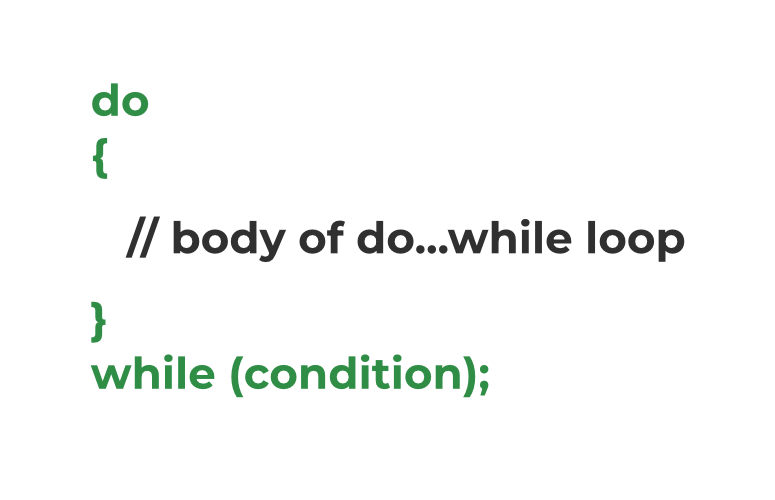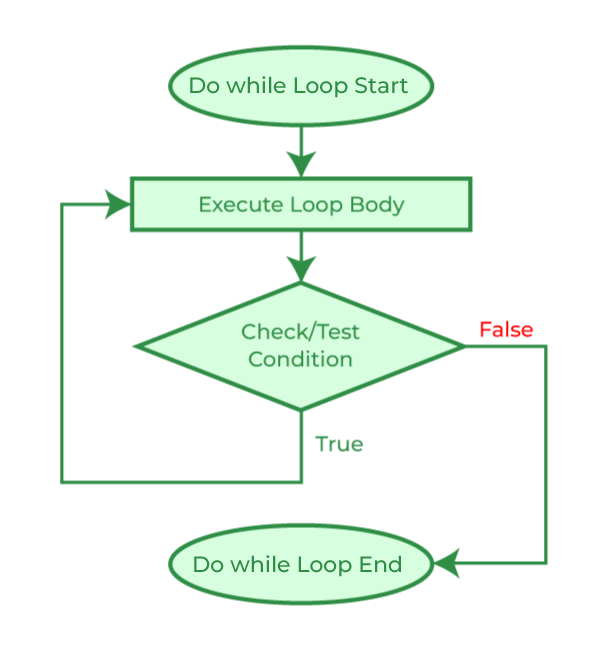# do…while Loop in C

Loops in C language are the control flow statements that are used to repeat some part of the code till the given condition is satisfied. The do-while loop is one of the three loop statements in C, the others being while loop and for loop. It is mainly used to traverse arrays, vectors, and other data structures.

## What is do…while Loop in C?

The do…while in C is a loop statement used to repeat some part of the code till the given condition is fulfilled. It is a form of an exit-controlled or post-tested loop where the test condition is checked after executing the body of the loop. Due to this, the statements in the do…while loop will always be executed at least once no matter what the condition is.

## Syntax of do…while Loop in C

```do {

// body of do-while loop

} while (condition);```

## How to Use do…while Loop in C

The following example demonstrates the use of do…while loop in C programming language.

## C

 `// C Program to demonstrate the use of do...while loop` `#include `   `int` `main()` `{`   `    ``// loop variable declaration and initialization` `    ``int` `i = 0;` `    ``// do while loop` `    ``do` `{` `        ``printf``(``"Geeks\n"``);` `        ``i++;` `    ``} ``while` `(i < 3);`   `    ``return` `0;` `}`

Output

```Geeks
Geeks
Geeks```

## How does the do…while Loop works?Syntax Structure of do while loop

The working of the do…while loop is explained below:

1. When the program control first comes to the do…while loop, the body of the loop is executed first and then the test condition/expression is checked, unlike other loops where the test condition is checked first. Due to this property, the do…while loop is also called exit controlled or post-tested loop.
2. When the test condition is evaluated as true, the program control goes to the start of the loop and the body is executed once more.
3. The above process repeats till the test condition is true.
4. When the test condition is evaluated as false, the program controls move on to the next statements after the do…while loop.

As with the while loop in C, initialization and updation is not a part of the do…while loop syntax. We have to do that explicitly before and in the loop respectively.

The flowchart below shows the visual representation of the flow of the do…while loop in C.

## C do…while Loop FlowchartFlowchart of do…while Loop in C

## Nested do…while Loop in C

As with other loops, we can also nest one do…while loop into another loop. It is demonstrated using the following C program.

## C

 `// C Program to demonstrate the nesting of do...while loop` `#include `   `int` `main()` `{`   `    ``// declaring loop variables` `    ``int` `i = 0, j;` `    ``int` `count = 0;`   `    ``// outer loop starts` `    ``do` `{` `        ``j = 0;`   `        ``// inner loop starts` `        ``do` `{` `            ``printf``(``"%d  "``, count++);` `            ``j++;` `        ``} ``while` `(j < 3);` `        ``// inner loop ends`   `        ``printf``(``"\n"``);` `        ``i++;` `    ``} ``while` `(i < 3);` `    ``// outer loop ends`   `    ``return` `0;` `}`

Output

```0  1  2
3  4  5
6  7  8  ```

To know more about nested loops in C, refer to this article – Nested Loops in C with Examples

## C

 `// C Program to demonstrate the do...while loop behaviour` `// when the condition is false from the start` `#include ` `#include `   `int` `main()` `{`   `    ``// declaring a false variable` `    ``bool` `condition = ``false``;`   `    ``do` `{` `        ``printf``(``"This is loop body."``);` `    ``} ``while` `(condition); ``// false condition`   `    ``return` `0;` `}`

Output

`This is loop body.`

As we can see, even when the condition is false at the start, the loop body is executed once. This is because in the do…while loop, the condition is checked after going through the body so when the control is at the start,

1. It goes through the loop body.
2. Executes all the statements in the body.
3. Checks the condition which turns out to be false.
4. Then exits the loop.

### Example 2. C Program to print Multiplication Table of N using do…while loop

The following example demonstrates the use of do…while loop for printing the multiplication table of N.

## C

 `// C Program to print multiplication table using do...while` `// loop` `#include `   `int` `main()` `{`   `    ``int` `N = 5, i = 1;`   `    ``do` `{` `        ``printf``(``"%d\tx\t%d\t=\t%d\n"``, N, i, N * i);` `    ``} ``while` `(i++ < 10);`   `    ``return` `0;` `}`

Output

```5    x    1    =    5
5    x    2    =    10
5    x    3    =    15
5    x    4    =    20
5    x    5    =    25
5    x    6    =    30
5    x    7    =    35
5    x    8    =    40
5    x    9    =    45
5    x    10    =    50```

## Difference between while and do…while Loop in C

The following table lists the important differences between the while and do…while Loop in C.

## Conclusion

In conclusion, the use of the only exit-controlled loop in C, the do…while loop is also to iterate a particular part of code but the way it works makes it different from entry-controlled loops such as the while loop and the for loop. It is useful in cases where we need to execute the statement inside the loop body at least once such as in traversing circular linked lists.

## FAQs on C do…while Loops

### 1. How many types of loops are there in C?

Ans: There are 3 types of loops in C language:

1. for Loop
2. while Loop
3. do…while Loop

### 2. What are the entry-controlled or pre-tested loops?

Ans: The entry-controlled loops or pre-tested loops are those loops in which the loop condition is checked before executing the body of the loop.

### 3. What are the exit-controlled or post-tested loops?

Ans: The exit-controlled loops or post-tested loops are those loops in which the program control comes to the body of the loop before checking the loop condition.

### 4. Which loop is guaranteed to execute at least once?

Ans: The do…while loop is guaranteed to execute the statements in the body of the loop at least once as it is a type of exit-controlled loop.

### 5. Can we skip braces in C do…while loop syntax if there is only one statement in the body?

Ans: No, we cannot skip braces in C do…while syntax even if there is only a single statement unlike while and for loop.

### 6. How to create an infinite loop in C using the do…while loop?

Ans: We can create an infinite loop in C by specifying a condition that will always be true as the loop condition. The below program demonstrates how to do that:

## C

 `// C Program to create a infinite loop using do...while loop` `// in C` `#include `   `int` `main()` `{` `    ``// infinite loop` `    ``do` `{` `        ``printf``(``"gfg "``);` `    ``} ``while` `(1); ``// always 1 ~ true`   `    ``return` `0;` `}`

Output

`gfg gfg gfg gfg gfg gfg gfg .... (infinite)`

### Related Articles:

Whether you're preparing for your first job interview or aiming to upskill in this ever-evolving tech landscape, GeeksforGeeks Courses are your key to success. We provide top-quality content at affordable prices, all geared towards accelerating your growth in a time-bound manner. Join the millions we've already empowered, and we're here to do the same for you. Don't miss out - check it out now!

Previous
Next